Home > CC2MN > Chapter cc27 > Lesson cc27.1.2 > Problem7-22

7-22.
1. Use the triangles below right to answer the questions that follow. Homework Help ✎

2.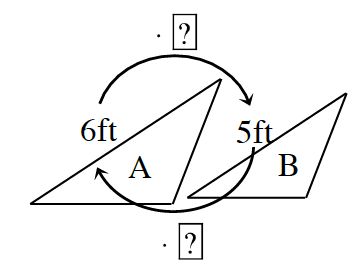1. What is the scale factor from A to B?

2. What is the scale factor from B to A?

3. What is the relationship of the scale factors?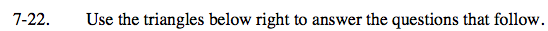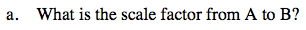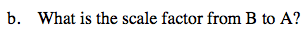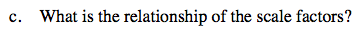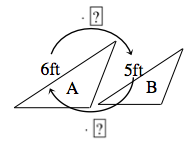What scale factor value times 6 ft would result in 5 ft? Consider the variable equation with x as the scale factor:
6x = 5
After isolating x, what do you get as the scale factor?

If the change from B to A is just the reverse of the change from A to B, what would the scale factor be? Create an expression like above if necessary.

The scale factors are reciprocals.

$\frac{5}{6}$